## 架构师之路 - 业务领域建模

|     |   0 浏览## 领域模型的概念及作用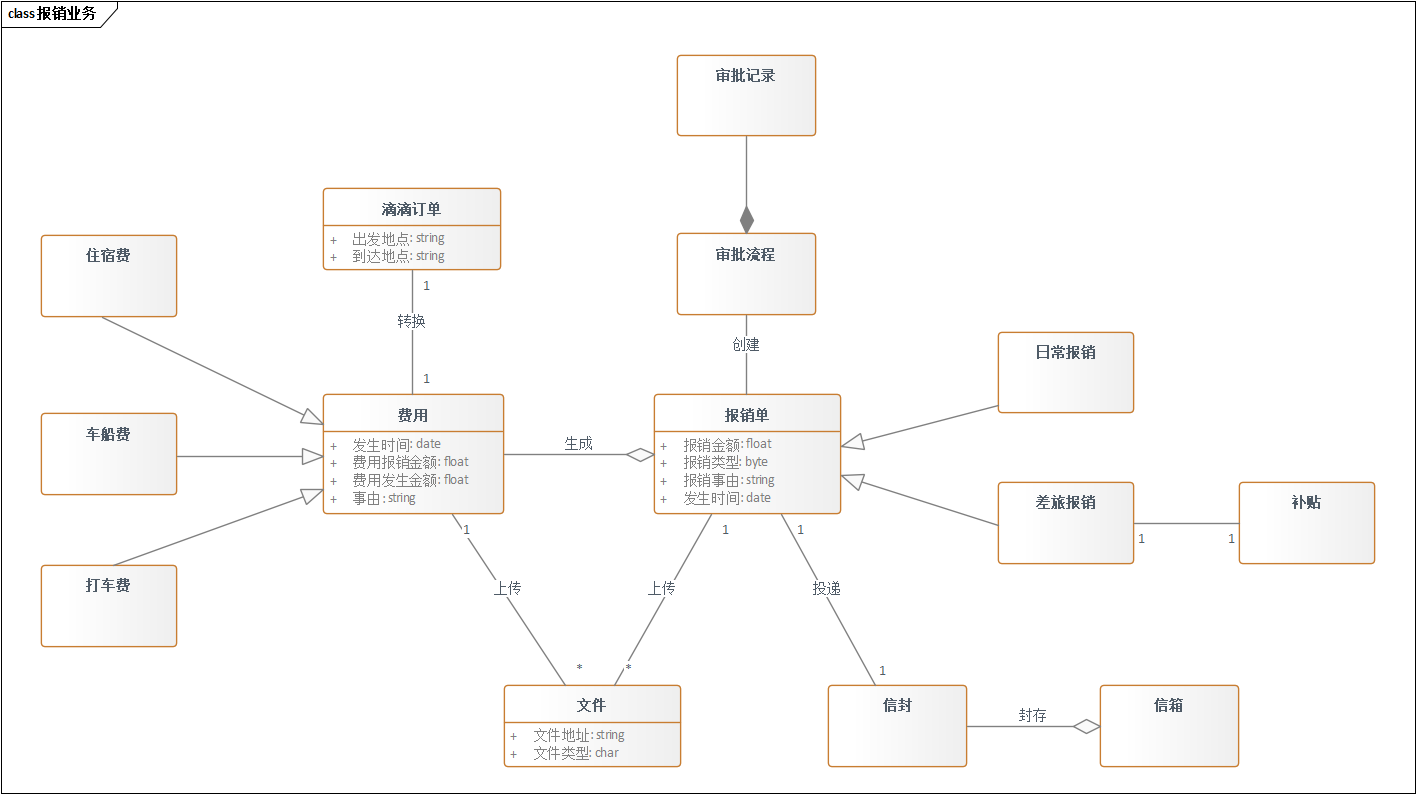1. 定义类的关键属性和关键行为；
2. 定义类与类之间的关联关系。

### 定义类的属性和行为

``````- 表示private
# 表示protected
~ 表示default,也就是包权限
+ 表示public
``````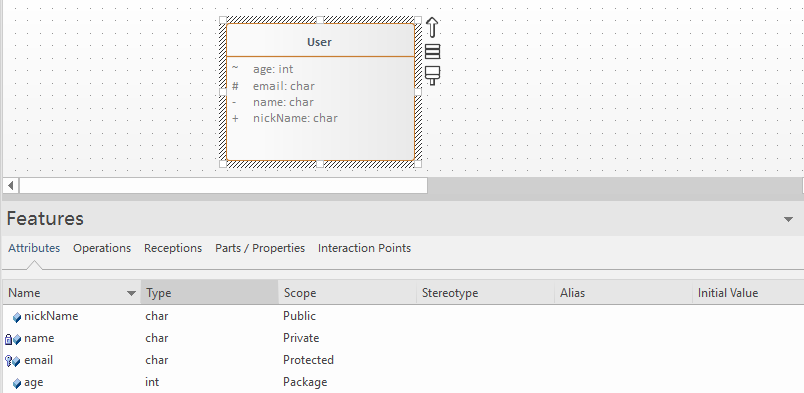### 定义类与类之间的交互关系

#### 泛化（Generalization）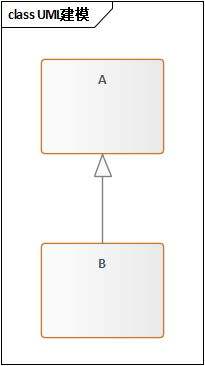``````public class A {

}

public class B extends A {

}
``````

#### 实现（Realization）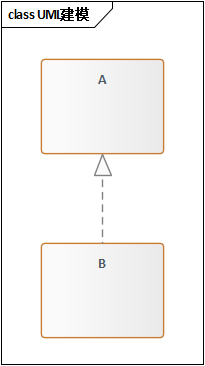``````public interface A {

}

public class B implements A {

}
``````

#### 聚合（Aggregation）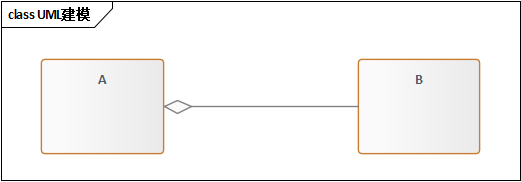``````public class A {
private B b;
public A(B b){
this.b = b;
}
}
``````

#### 组合(Composition)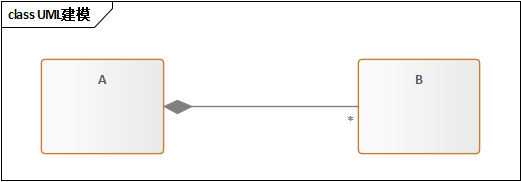``````public class A {
private B b;
public A () {
this.b = new B();
}
}
``````

#### 关联（Association)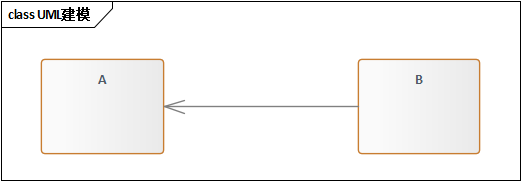``````public class A {
private B b;
public A(B b){
this.b = b;
}
}
``````

``````public class A {
private B b;
public A () {
this.b = new B();
}
}
``````

#### 依赖(Dependency)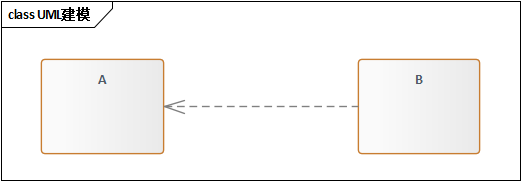``````public class A {
private B b;
public A(B b){
this.b = b;
}
}
``````

``````public class A {
private B b;
public A () {
this.b = new B();
}
}
``````

``````public class A {
public void func(B b)
...
}
}
``````

### 模型简化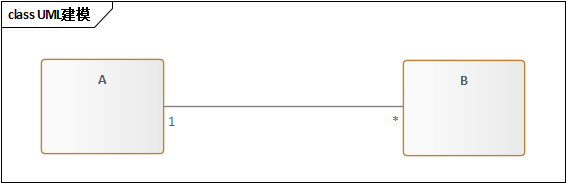### 小结

• 将待开发系统也放在领域模型里面
待开发系统要不要出现在领域模型中取决于你的业务离开待开发的系统能不能玩的转。举个例子：如果开发的是共享单车的信息系统，共享单车离开信息系统肯定玩不转，所以这时候信息系统需要出现在领域模型。
• 概念划分不清，关系没有画到位
比如属性画成了类，继承关系搞错